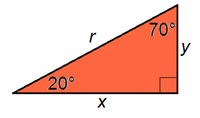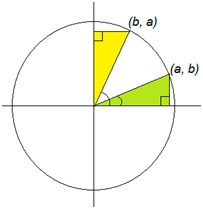# Trigonometric Identities - Day 2 of 2

## Objective

SWBAT simplify expressions and prove trigonometric identities.

#### Big Idea

Identifying what makes a trig expression confusing will keep you focused on what needs to be simplified.

## Launch and Explore

10 minutes

Today we will continue to look at trigonometric identities and the reasons that they are true. To start, I plan to have students refresh the collective memory of the class with respect to the identities that we discussed yesterday. We will brainstorm a list of identities. As we do, I will encourage students to look at their summary sheet of the identities and discuss (with their table) why these relationships are true.

During today’s lesson we will focus on why the co-function properties are true. To get started, I write “sin 70° = cos ______” on the board and ask students to fill in an angle measure that will make the statement true. I stress that whatever answer they choose, they should be able to produce a diagram or explanation to show it is true. I give students about 7 minutes to work on this. While they are working, I keep an eye out for students who get the answer but cannot verify that it is true. If a student only uses their calculator to verify, I will ask them how they would know it is true without being to check on a calculator.

## Share

15 minutes

I expect that some of my students may draw a diagram such as the one below to show that cos 20° = x/r and sin 70° = y/r. If so, I will choose a student who drew this and have them share their diagram. Then, I will tell the rest of my students to discuss at their table why the diagram proves the relationship. Finally, I will choose a different student than the one who originally drew this diagram to explain how it represents the relationship sin 70° = cos 20°.Another explanation that I expect to see is represented by the diagram below. Students may notice that if 70° and 20° are graphed in the unit circle, the triangles are congruent and the x-value of the 20° triangle is the same as the y-value of the 70° triangle. Again, I will choose a student who drew this and have them show the class. Then, I will ask a different student to explain how this diagram can be used.If I don’t get these two representations from my students, I will present both diagrams and give students some time to think how they prove that sin 70° = cos 20°. At this stage of this unit, both explanations are important milestones for students to visit and be able to refer back to.

To continue our progress, I will ask students to fill in the blank again for “cos 33° = sin ______”. Again, I will give a few minutes to work on the problem. At this point, students may begin with the conjectire that you can always subtract the cosine angle from 90° to get the sine angle. If so, I will label this as a conjecture and see if it always works. I will allow time for my students to try a few on their own. For example, I will ask my students to confirm if it works for an angle greater than 90° - does cos 120° = sin (-30°)?

After coming up the relationship sin x = cos(90° - x), I will graph both functions on Desmos and verify that the value of both graphs at every x-value is the same. Students may wonder if this would also work for the other trig functions. To satisfy their curiosity, we may explore these from a graphical perspective to see if they work.

## Summarize

25 minutes

Now we are going to use the new identity to prove the statement: tan x [ cos(90° - x) + cot x cos x] = sec x . Like yesterday, I want students to focus in on what makes this equation difficult so they will know what we have to focus on simplifying. For this one, there are multiple trig functions and their arguments are not the same (more on this in the video below).

I plan to have them start with the arguments – how can we rewrite cos(90° - x) so that it has an argument of just x like the rest of the expressions? Then work on getting rid of trig functions – writing everything in terms of sine and cosine is a great idea and a strategy that will help them out often. After discussing these hints, have them try it out to see if they can simplify the expression to equal sec x. After giving them a few minutes, have a student present their work to the class. Bring up the fact that students can prove things in different ways; there is not one unique method to get the left side of the identity to equal the right side.

Attached is an assignment where students can get some practice with proving trig identities. There are a lot of problem on this sheet, so I would assign #2, 4, 5, 10, 12, 15, and 16. The rest can be used as practice for if students feel they need it.